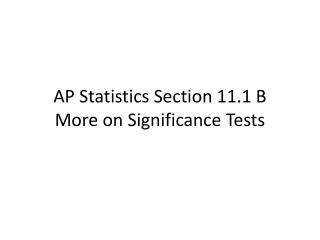Download PresentationAP Statistics Section 11.1 B More on Significance Tests

# AP Statistics Section 11.1 B More on Significance Tests - PowerPoint PPT PresentationDownload Presentation## AP Statistics Section 11.1 B More on Significance Tests

- - - - - - - - - - - - - - - - - - - - - - - - - - - E N D - - - - - - - - - - - - - - - - - - - - - - - - - - -
##### Presentation Transcript

1. AP Statistics Section 11.1 BMore on Significance Tests

2. Conditions for Significance TestsThe three conditions that should be satisfied before we conduct a hypothesis test about an unknown population mean or proportion are the same as they were for confidence intervals: 1. _______ from the population of interest.2. Distribution of must be ________________ For : _________________________________ For : ________________________3. _________________________ If sampling w/o replacement ___________

3. Example 1: Check that the conditions from the paramedic example in section 11.1 A are met.SRS:Normality of :Independence:

4. Test StatisticsA significance test uses data in the form of a test statistic. The following principles apply to most tests: (1) the test statistic compares the value of the parameter as stated in the __ to an estimate of the parameter from the sample data. (2) values of the estimate far from the parameter value in the direction specified by the alternativehypothesis give evidence _____________ (3) to assess how far the estimate is from the parameter, standardize the estimate.

5. In many common situations, the test statistic has the form:test statistic = -----------------------------------------

6. Because the result is over two standard deviations below the hypothesized mean 6.7, it gives good evidence that the mean RT this year is not equal to 6.7 minutes, but rather, less than 6.7 minutes.

7. The probability, computed assuming __________, that the observed sample outcome would take a value as extreme as or more extreme than that actually observed is called the __________ of the test.

8. The smallerthe P-value is, the strongerthe evidence is against provided by the data.

9. Example 3: Let’s go back to our paramedic example. The P-value is the probability of getting a sample result at least as extreme as the one we did ( = 6.48) if were true. In other words, the P-value is calculated assuming . We just found the z-score for this exact situation, so using Table A or our calculator, this P-value is _______. So ifis true, and the mean RT this year is still 6.7 minutes, there is about a _____ chance that the city manager would obtain a sample of 400 calls with a mean RT of 6.48 minutes or less. The small P-value provides strong evidence against and in favor of the alternative minutes.

10. Example 4: Suppose we know that differences in job satisfaction scores in Example 3 of section 11.1 A follow a Normal distribution with standard deviation . If there is no difference in job satisfaction between the two work environments, the mean is _______. Thus H0: ________. The Ha says simply “there is a difference,” thus Ha:________. Data from 18 workers gave 17. That is, these workers preferred the self-paced environment on average. Find the p-value for this situation and interpret it.

11. A p-value of .2302 indicates that 23.02% of the time we will get a sample where is at least as big as 17 when . An outcome that would occur this often when is not good evidence that .

12. Statistical SignificanceWe can compare the P-value with a fixed value that we regard as decisive. This amounts to announcing in advance how much evidence against we will insist on. The decisive value of P is called the significance level. We write it as ____, the Greek letter alpha. If the P-value , we say that the data are

13. Example 5: Back to the paramedic example. We found the P = 0.0139. The result is statistically significant at the .05 level since P < ____ but it is not significant at the .01 level since P > ____“Significant” in the statistical sense does not mean “_____________.” It means simply “not likely to happen just by _________.”

14. Interpreting Results in ContextThe final step in performing a significance test is to draw a conclusion about the competing claims you were testing. As with confidence intervals, your conclusion should have a clear connection to your calculations and should be stated in the context of the problem. These are called the 3C’s.

15. In significance testing, there are two accepted methods for drawing conclusions:

16. In examples 3 and 4 of this section we simply stated the p-value and interpreted it in the context of the problem.

17. In example 5, we went on to determine if the data was statistically significant be comparing our P-value to our significance level . We can either _______ or _______________ the Ho based on whether our result is statistically significant at a given significance level.

18. Warning: if you are going to draw a conclusion based on statistical significance, then the significance level should be stated before the data are produced.

19. Example 6: For the paramedic example, we calculated the P-value to be 0.0139. If we were using an significance level, we would _____ minutes ( ________ ) since ______ ( __________ ). It appears that the mean response time to all life-threatening calls this year is less than last year’s average of 6.7 minutes ( ______ ).

20. Finally, stating a P-value is more informative than simply giving a “reject” or “fail to reject” conclusion at a given significance level. For example, a P-value of 0.0139 allows us to reject at the level. But the P-value, 0.0139 gives us a better sense of how strong the evidence against is. The P-value is the smallest level at which the data are significant.